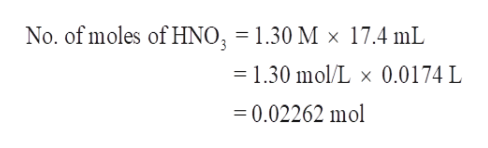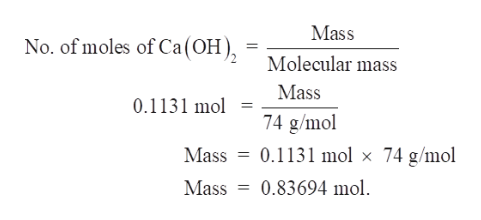# How many grams of solid calcium hydroxide are needed to exactly neutralize 17.4 mL of a 1.30 M nitric acid solution ? Assume that the volume remains constant

Question
4 views

How many grams of solid calcium hydroxide are needed to exactly neutralize 17.4 mL of a 1.30 M nitric acid solution ? Assume that the volume remains constant

check_circle

Step 1

The balanced chemical equation of Ca(OH)2 and HNO3 is –

Step 2

Since, 2 moles of HNO3 reacts with 1 mole of Ca(OH)2.

Therefore, 1 moles of HNO3 reacts with 0.5 mole of Ca(OH)2.

To calculate the amount of solid Ca(OH)2 required to neutralise 17.4 ml of a 1.30 M HNO3, we will first calculate the no. of moles of Ca(OH)2  and HNO3.help_outlineImage TranscriptioncloseNo. of moles of HNO = 1.30 M x 17.4 mL 1.30 mol/L x 0.0174 L 0.02262 mol fullscreen
Step 3

Now as per the balanced equation, I mole of HNO3 reacts with 0.5 mole of Ca(OH)2.

Hence, 0.02262 mol of HNO3 reacts with 0.5 mole of Ca(OH)2 gi...help_outlineImage TranscriptioncloseMass No. of moles of Ca(OH) Molecular mass Mass 0.1131 mol 74 g/mol = 0.1131 mol x 74 g/mol Mass 0.83694 mol Mass fullscreen

### Want to see the full answer?

See Solution

#### Want to see this answer and more?

Solutions are written by subject experts who are available 24/7. Questions are typically answered within 1 hour.*

See Solution
*Response times may vary by subject and question.
Tagged in

### Chemistry